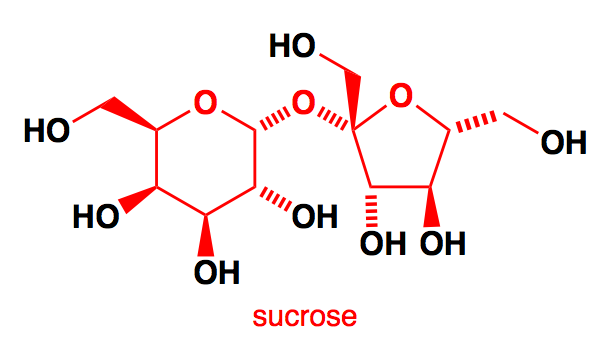QuestionAnswers

# The number of moles of acetic anhydride $\left( {A{c_2}O} \right)$ needed to react completely with sucrose is:Verified
131.1k+ views
Hint: In this problem firstly, we will put light on a very basic yet very important topic of chemistry. In this question we will learn about Biomolecules and Disaccharides and we will be learning all the related information on them which will help us in approaching our answer. Below here these concepts are explained properly.

$i.e$ monosaccharides combine to form a disaccharide. Disaccharides are those carbohydrates that on hydrolysis with acids or enzymes give two molecules of monosaccharides which can either be the same or different. Monosaccharide units are joined together via a linkage called glycosidic linkage.
It has been observed that the structure of Sucrose, it can be seen that it has .$8 - OH$ groups and each $- OH$ reacts with the acetic anhydride. So, $8\,moles$ of acetic anhydride are required from one mole of sucroseNote: Sucrose is found in all photosynthetic plants. It is commonly obtained from sugarcane and sugar beets via an industrial process. The molecular formula of sucrose is ${C_{12}}{H_{22}}{O_{11}}$ . If sucrose goes through acid catalyzed hydrolysis it produces one mole of D – Glucose and one mole of D- Fructose. Sucrose is a non- reducing sugar. Sucrose structure is combined at the hemiacetal oxygen and does not have a free hemiacetal hydroxide.